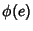## Haupt-Exponent

The smallest exponentfor which, whereandare given numbers, is the haupt-exponent of(mod). The number of bases having a haupt-exponentis, whereis the Totient Function. Cunningham (1922) published the haupt-exponents for primes to 25409 and bases 2, 3, 5, 6, 7, 10, 11, and 12.